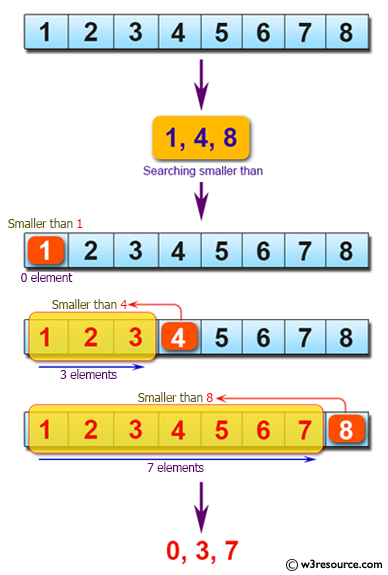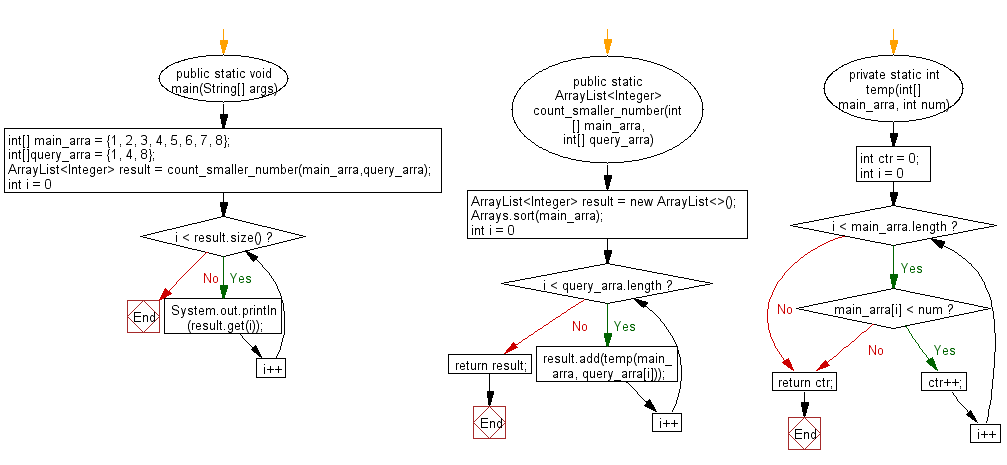﻿ Java programming exercises: Get the number of element that are smaller than the number of another - w3resource# Java Exercises: Get the number of element that are smaller than the number of another

## Java Basic: Exercise-172 with Solution

Write a Java program to get the number of element in a given array of integers that are smaller than the integer of another given array of integers.

Pictorial Presentation:Sample Solution:

Java Code:

``````import java.util.ArrayList;
import java.util.Arrays;
public class Solution {
public static void main(String[] args) {
int[] main_arra = {1, 2, 3, 4, 5, 6, 7, 8};
int[]query_arra = {1, 4, 8};
ArrayList < Integer > result = count_smaller_number(main_arra, query_arra);
for (int i = 0; i < result.size(); i++) {
System.out.println(result.get(i));
}
}
public static ArrayList < Integer > count_smaller_number(int[] main_arra, int[] query_arra) {
ArrayList < Integer > result = new ArrayList < > ();
Arrays.sort(main_arra);
for (int i = 0; i < query_arra.length; i++) {
}
return result;
}
private static int temp(int[] main_arra, int num) {
int ctr = 0;
for (int i = 0; i < main_arra.length; i++) {
if (main_arra[i] < num) {
ctr++;
} else {
break;
}
}
return ctr;
}
}
```
```

Sample Output:

```0
3
7
```

Flowchart:Java Code Editor:

What is the difficulty level of this exercise?

﻿

## Java: Tips of the Day

Parsing dates:

```import java.io.*;
import java.util.*;
import java.text.*;

String s = "2001/09/23 14:39";

SimpleDateFormat formatter = new SimpleDateFormat ("yyyy/MM/dd H:mm");
Date d = formatter.parse(s, new ParsePosition(0));
```